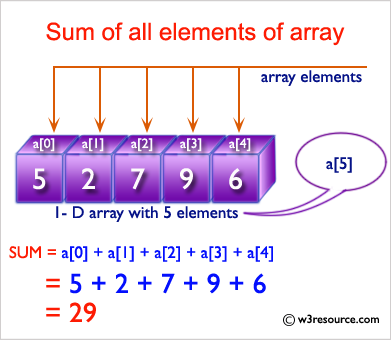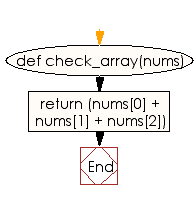﻿ Ruby Array exercises: Compute the sum of all the elements - w3resource# Ruby Array Exercises: Compute the sum of all the elements

## Ruby Array: Exercise-9 with Solution

Write a Ruby program to compute the sum of all the elements. The array length must be 3 or more.Ruby Code:

``````def check_array(nums)
return (nums + nums + nums)
end

print check_array([1, 2, 5]),"\n"
print check_array([1, 2, 3]),"\n"
print check_array([1, 2, 4])
``````

Output:

```8
6
7
```

Flowchart:Ruby Code Editor: